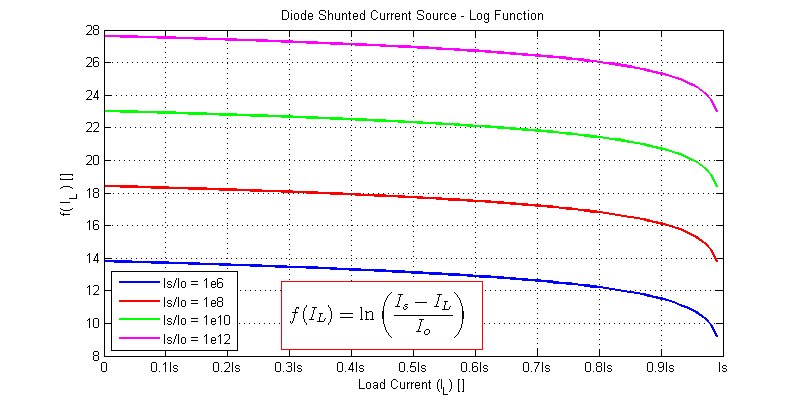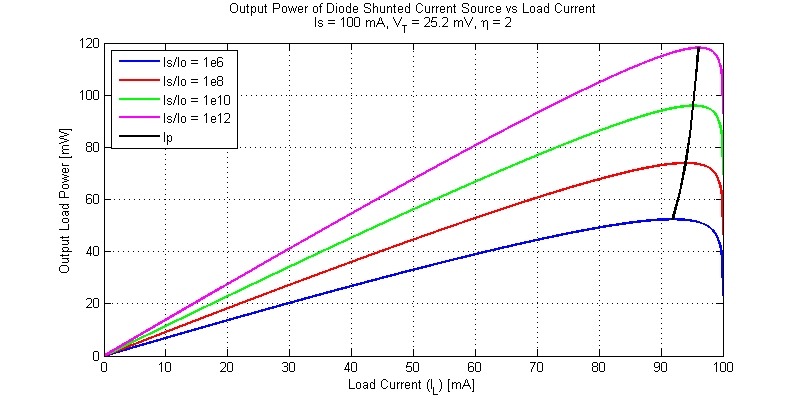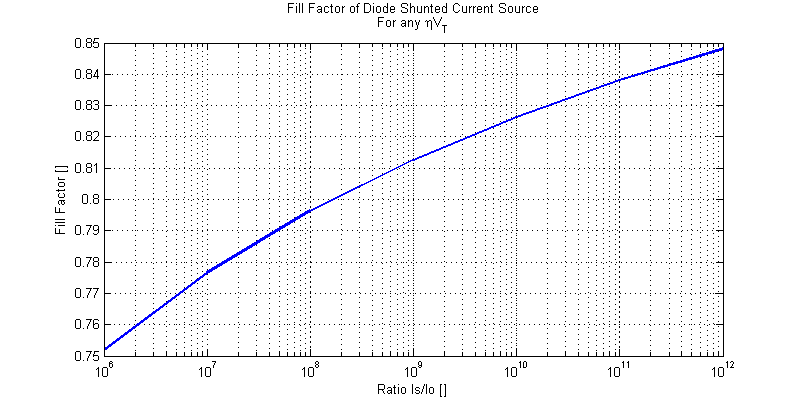# Maximum Power Point of Diode Shunted Current Source

In this post, the maximum power point (MPP) of a diode shunted current source will be assessed and modeled. A solar cell in its simplest description is merely a semiconductor diode. When the cell’s junction is illuminated, a photo current $$Is$$ is produced. A basic schematic model of an ideal solar cell is shown in the figure below.For diode forward voltages $$V_D$$ greater than a few $$\eta V_T$$, such as 100 mV, the diode equation is approximately equal to,

$$I_D \simeq I_o exp\left( \dfrac{V_D}{\eta V_T} \right)$$

Applying KCL at the output node of the schematic above yields,

$$I_D + I_L = I_S$$

$$I_D = I_S – I_L$$

The forward voltage of the diode can be determined as,

\begin{align*}
V_D &= \ln \left( \dfrac{I_D}{I_o} \right) \eta V_T \\
V_D &= \ln (I_S – I_L)\eta V_T -\ln (I_o)\eta V_T
\end{align*}

The output load power is equal to,

$$P_L = V_L \cdot I_L$$

Since the output terminals are in parallel to the shunt diode’s terminals the output load voltage is identical to the diode’s forward voltage,

$$V_L = V_D$$

Hence, the output load power as a function of load current becomes,

$$P_L = \ln( I_S – I_L )\eta V_T I_L – \ln(I_o)\eta V_T I_L$$

$$\dfrac{\partial P_L}{\partial I_L} = \dfrac{\eta V_TI_L(-1)}{I_S-I_L} + \ln(I_S-I_L)\eta V_T – \ln(I_o)\eta V_T$$

We can determine the MPP as the the inflection point where $$\partial P_L/\partial I_L$$ equals 0, as,

\begin{align*}
\left| \dfrac{\partial P_L}{\partial I_L} \right|_{I_L=I_P} &= 0 \\
\ln \left( \dfrac{I_S – I_P}{I_o} \right)\eta V_T &= \dfrac{ I_P \eta V_T}{I_S – I_P} \\
\end{align*}

We then arrive at the following expression for the MPP load current,

$$\ln \left( \dfrac{I_S – I_P}{I_o} \right) = \dfrac{I_P}{I_S – I_P} \tag{EQ.1}$$

The solution for the load current $$I_L$$ which yields the maximum output load power is only a function of the current source $$I_S$$ and the diode’s saturation current $$I_o$$. The solution of $$\ln(ax)=bx+c$$ is non-trivial. Hence, we will attempt to find an approximation for the natural log function. A plot left-hand term of EQ.1 is shown in the figure below.Here to simplify the solution we will begin by assuming the maximum power point occurs at 0.9 I_s (10% less than a short circuit condition). The natural logarithm can then be approximated as,

$$\ln \left( \dfrac{I_S – 0.9I_S}{I_o} \right) = \ln \left( \dfrac{0.1I_S}{I_o} \right)$$

Substitution our approximated log term yields,

$$\ln \left( \dfrac{0.1I_S}{I_o} \right) = \dfrac{ I_P}{I_S – I_P}$$

The approximate MPP load current is then,
$$I_P = I_S\left( \dfrac{\ln(0.1I_S/I_o)}{1+\ln(0.1I_S/I_o)} \right)$$

The table below provides the approximate MMP load current for a diverse range of source currents $$I_S$$ and diode saturation currents $$I_o$$.

Approximate Solution for MPP Load Current
$$I_S/I_o$$ MPP Current $$I_P$$
$$10^6$$ $$0.920 I_S$$
$$10^7$$ $$0.933 I_S$$
$$10^8$$ $$0.942 I_S$$
$$10^9$$ $$0.949 I_S$$
$$10^{10}$$ $$0.954 I_S$$
$$10^{11}$$ $$0.958 I_S$$
$$10^{12}$$ $$0.962 I_S$$

The output power of a diode shunted current for an assortment of load and source conditions is shown in the figure below.Even when the ratio $$I_S/I_o$$ varies by over 6 orders of magnitude the MPP load current is only perturbed from $$0.919I_S$$ to $$0.961I_S$$.

A plot for diode ideality factor $$\eta = 2$$ is shown in the figure below.As shown in the analysis above, the MPP load current is not a function of $$V_T$$ or $$\eta$$.## Solar Perspective

The fill factor of a solar cell is defined as,

$$FF = \dfrac{V_{mp}I_{mp}}{V_{oc}I_{sc}}$$

We have seen that for a diode shunted current source the MPP load current is roughly 92% to 96% of the short-circuit current. Hence the ratio $$I_{mp}/I_{sc}$$ is known and relatively constant over a diverse range of illumination currents and junction saturation currents.

During open-circuit conditions, all of the generated photo current is conducted by the shunt diode. At the maximum power point the conducted current through the diode declines to 4% to 8% of the short-circuit current (the rest of the photo current is delivered to the load). We can solve the decline in forward voltage as,

$$\Delta V_D = \eta V_T \ln\left( \dfrac{I_S}{I_S – I_P} \right)$$

As an illustration consider,

$$\eta = 1, \;\;\; V_T = 25.2 \text{ mV},\;\;\; I_S = 100 \text{ mA}, \;\;\;I_o = 100 \text{ pA}$$

The MPP load current is then approximately,

$$I_P \simeq 0.95 I_S$$

$$\Delta V_D = 25.2 \ln( 1/0.05 ) = 25.2 \ln(20) = 75.5 \text{mV}$$

The current source’s open circuit voltage is,

$$V_{oc} = 25.2 \ln( 100 \text{ mA} / 100 \text{ pA} ) = 522.2 \text{ mV}$$

$$V_{mp} = 25.2 \ln( I_D / 100 \text{ pA}) = 25.2\ln(5 \text{ mA}/100 \text{ pA}) = 446.7 \text{ mV}$$

Finally we can determine the fill factor for this example as,

$$FF = \dfrac{(446.7)(0.95)}{(552.1)(1)} = 0.812$$

$$P_{max} = (0.812)V_{oc}I_{sc}$$

The take away from all of this, is, that the load current for maximum output power is approximately equal to 95% of the short-circuit current. Since the ratio of $$I_{mp}/I_{sc}$$ is approximately constant, the decline in output voltage $$\Delta V$$ from $$V_{oc}$$ to $$V_{mp}$$ is also a constant number of $$(\eta V_T)$$ ‘s.

One could loosely approximate the fill factor of a single solar cell as,

$$FF \simeq \left(\dfrac{V_{oc} – 75\text{ mV}}{V_{oc}}\right) (0.95)$$

Which clearly indicates that the larger the open circuit voltage (either by larger photo current or by a smaller saturation current) the higher the fill factor. A plot of fill factor for a wide range of photo and diode saturation currents is shown in the figure below.This site uses Akismet to reduce spam. Learn how your comment data is processed.